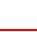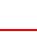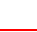Skill 6C Fact Families: Writing Here, your student is to make the fact family for the numbers 5, 9 and 14. As you recall, a fact family is simply putting the same three numbers into a different order to make four TRUE number sentences. Three numbers always have a fact family of four different number sentences. Two are addition and two are subtraction. So the fact family for 4, 9 and 14 is: 5+9=14 9+5=14 14-9=5 14-5=9 Use the numbers 5, 9 and 14 to make their complete fact family.+=5+=9 14-=-=